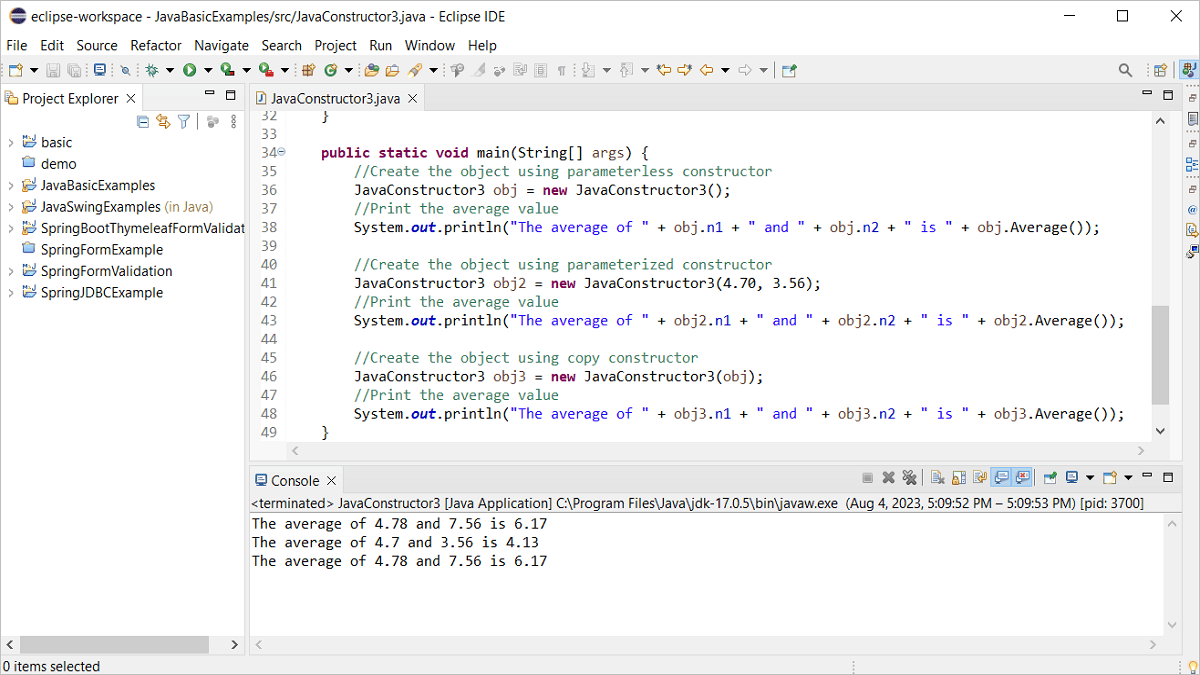# FahmidasClassroom

Learn by easy stepsDifferent types of constructors can be defined in Java to do the initialization tasks. The way of defining parameterless constructor, parameterized constructor, and copy constructor has been shown in this tutorial.

## Example-1: Parameterless constructor

Create a Java file with the following code that will use the parameterless constructor to initialize the class variables and print the summation of the variables.

``````public class JavaConstructor {
//Declare two private variables
private int n1, n2;

//Define the parameterless constructor method
public JavaConstructor() {
n1 = 16;
n2 = 45;
}

//Define class method
{
int result = n1 + n2;
return result;
}

public static void main(String[] args) {
//Create the object
JavaConstructor obj = new JavaConstructor();
//Print the summation value
System.out.println("The sum of " + obj.n1 + " and " + obj.n2 + " is " + obj.add());
}

}``````

Output:

## Example-2: Parameterized constructor

Create a Java file with the following code that will use the parameterized constructor to initialize the class variables and print the multiplication of the variables.

``````
public class JavaConstructor2 {
//Declare two private variables
private int n1, n2;

//Define the parameterized constructor method
public JavaConstructor2(int a, int b) {
n1 = a;
n2 = b;
}

//Define class method
public int Multiply()
{
int result = n1 * n2;
return result;
}

public static void main(String[] args) {
//Create the object
JavaConstructor2 obj = new JavaConstructor2(2, 100);
//Print the multiplication value
System.out.println("The multiplication of " + obj.n1 + " and " + obj.n2 + " is " + obj.Multiply());
}

}
``````

Output:

## Example-3: Multiple constructors

Create a Java file with the following code that will use the copy constructor to initialize the class variables and print the average of the variables.

``````import java.text.DecimalFormat;

public class JavaConstructor3 {
//Declare two private variables
private double n1, n2;

//Define the parameterless constructor method
public JavaConstructor3() {
n1 = 4.78;
n2 = 7.56;
}

//Define the parameterized constructor method
public JavaConstructor3(double a, double b) {
n1 = a;
n2 = b;
}

//Define the copy constructor method
public JavaConstructor3(JavaConstructor3 obj) {
n1 = obj.n1;
n2 = obj.n2;
}

//Define class method
public double Average()
{
double result = (n1 + n2)/2;
DecimalFormat ft = new DecimalFormat("#.##");
return Double.valueOf(ft.format(result));
//return result;
}

public static void main(String[] args) {
//Create the object using parameterless constructor
JavaConstructor3 obj = new JavaConstructor3();
//Print the average value
System.out.println("The average of " + obj.n1 + " and " + obj.n2 + " is " + obj.Average());

//Create the object using parameterized constructor
JavaConstructor3 obj2 = new JavaConstructor3(4.70, 3.56);
//Print the average value
System.out.println("The average of " + obj2.n1 + " and " + obj2.n2 + " is " + obj2.Average());

//Create the object using copy constructor
JavaConstructor3 obj3 = new JavaConstructor3(obj);
//Print the average value
System.out.println("The average of " + obj3.n1 + " and " + obj3.n2 + " is " + obj3.Average());
}

}
``````

Output:

## Exercise:

Create a Java class with the parameterized constructor that will take customer account information of a bank account (customer id, account type, and opening balance). The class will contain three methods to add amount, deduct amount and display the current account information. Write code to check the following conditions at the time of initializing the customer data and deducting amount from the balance.

A. Opening balance must not be less than 1000 taka.

B. Current balance can’t be less than 500 taka.